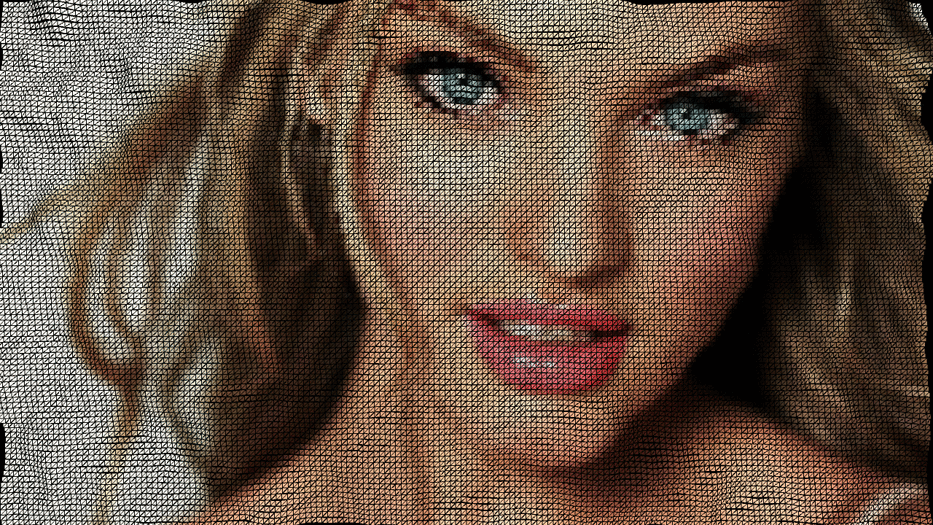# Mapped image to animated gridIn the following explorations, we take the code of “Mesh trickery” and mix it up with some other code to add extra functionality. The idea was to create the same effect, but mapping an image into the distorted grid. I found a really helpful library that allowed me to do this. Also, changing values in the rotating axis angle yielded some interesting results as well as animating the ondulations in different directions.

See code below:

```import generativedesign.*; ```
``` PImage img; color[] colors; String sortMode = null; float[][] terrain; float flying =0; int cols, rows; int scl = 2; int w = 1280+200; int h =720+200; void setup() { size(1280, 720, P3D); colorMode(HSB, 360, 100, 100, 100); noStroke();```

```cols = w/scl; rows = h/scl;```

```noCursor(); img = loadImage("img3.jpg"); frameRate(30); } void InitTerrainArr() { terrain = new float[cols][rows]; flying -=0.04; float yoff = flying; for (int y = 0; y<rows; y++) { //flying +=0.0001; float xoff = 0; for (int x = 0; x<cols; x++ ) { terrain[x][y] = map(noise(xoff, yoff), 0, 1, -50, 100); xoff += 0.1; } yoff += 0.1; } } void draw() { background(0); stroke(255); InitTerrainArr(); //rotateAxis(PI/6); int tileCount = width / max(mouseX, 5); //int tileCount = 200; float rectSize = width / float(tileCount); // get colors from image int i = 0; colors = new color[tileCount*tileCount]; for (int gridY=0; gridY<tileCount; gridY++) { for (int gridX=0; gridX<tileCount; gridX++) { int px = (int) (gridX * rectSize); int py = (int) (gridY * rectSize); colors[i] = img.get(px, py); i++; } } // sort colors if (sortMode != null) colors = GenerativeDesign.sortColors(this, colors, sortMode); // draw grid i = 0; for (int gridY=0; gridY<tileCount; gridY++) { beginShape(TRIANGLE_STRIP); for (int gridX=0; gridX<tileCount; gridX++) { fill(colors[i]); stroke(0); //rect(gridX*rectSize, gridY*rectSize, rectSize, rectSize); //vertex(gridX*rectSize,gridY*rectSize,terrain[gridX][gridY]); //vertex(gridX*rectSize,(gridY+1)*rectSize,terrain[gridX][gridY]);```
```vertex(gridX*rectSize, gridY*rectSize, terrain[gridX][gridY]); vertex(gridX*rectSize, (gridY+1)*rectSize, terrain[gridX][gridY+1]); ```
```i++; } endShape(); }```

```} void rotateAxis(float angle) { //translate(width/2,height/2); translate(900, 0); float a = angle; rotateX(a); translate(-800, 10); }```